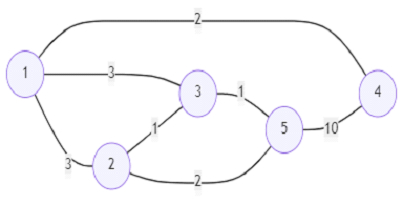# Program to find number of restricted paths from first to last node in Python

Suppose we have one undirected weighted connected graph. The graph has n nodes and they are labelled from 1 to n. A path from start to end is a sequence of nodes like [z0, z1, z2, ..., zk] here z0 is start node and zk is end node and there is an edge between zi and zi+1 where 0 <= i <= k-1. The distance of a path is the sum of the weights values on the edges of the path. Let dist(x) denotes the shortest distance from node n and node x. A restricted path is a special path that also satisfies that dist(zi) > dist(zi+1) where 0 <= i <= k-1. So, we have to find the number of restricted paths from node 1 to node n. If the answer is too large then return answer modulo 10^9 + 7.

So, if the input is likethen the output will be 3 because there are three restricted paths (1,2,5), (1,2,3,5), (1,3,5).

To solve this, we will follow these steps −

• graph := adjacency list of the graph made from given edge list

• paths := an array of size (n+1) and filled with 0

• paths[n] := 1

• dists := an array of size (n+1) and filled with -1

• q := a queue, and insert (0, n) initially

• while q is not empty, do

• (dist, node) := front element of q and remove it from q

• if dists[node] is not same as -1, then

• go for next iteration

• dists[node] := dist

• for each adjacent node v and weight w of graph[node], do

• if dists[v] is same as -1, then

• insert (dist + w, v) into q

• otherwise when dists[v] < dists[node], then

• paths[node] := paths[node] + paths[v]

• if node is same as 1, then

• return paths[node] mod 10^9+7

• return 0

## Example

Let us see the following implementation to get better understanding −

from collections import defaultdict
from heapq import heappop, heappush
def solve(n, edges):
graph = defaultdict(dict)
for u, v, w in edges:
graph[u][v] = w
graph[v][u] = w

paths =  * (n+1)
paths[n] = 1
dists = [-1] * (n+1)
q = [(0, n)]

while q:
dist, node = heappop(q)
if dists[node] != -1:
continue

dists[node] = dist
for v, w in graph[node].items():
if dists[v] == -1:
heappush(q, (dist + w, v))
elif dists[v] < dists[node]:
paths[node] += paths[v]

if node == 1:
return paths[node] % (10**9 + 7)

return 0

n = 5
edges = [(1,2,3),(1,3,3),(2,3,1),(1,4,2),(5,2,2),(3,5,1),(5,4,10)]
print(solve(n, edges))

## Input

5,[(1,2,3),(1,3,3),(2,3,1),(1,4,2),(5,2,2),(3,5,1),(5,4,10)]


## Output

3